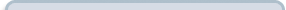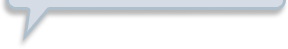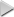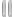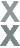# Fan Appreciation Night, Part 2

###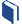Resources for this lesson: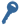Key Terms

Union

> Glossary> Calculator Resources> Teacher Resources: Instructional NotesThink of each event as a circle or group.  For mutually exclusive events, the events would be represented with non-overlapping circles.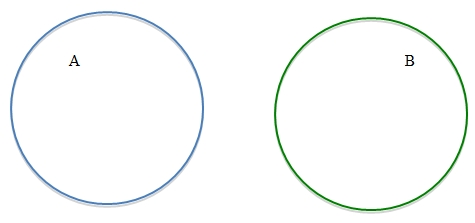To calculate probabilities of mutually exclusive events, the probability of either event occurring is simply the sum of the probabilities of each individual event.  So,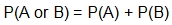. The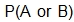is known as finding the probability of the union of A and B, and may also be written as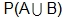.

For the first drawing, the probability of Allyson or Andrew winning would be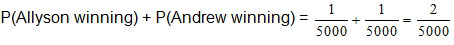.

##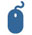Check Your Understanding